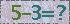2019年JOS入选“中国科技期刊卓越行动计划”
2020年11月JOS被EI数据库收录
J. Semicond. > Volume 35 > Issue 7 > Article Number: 075004

# A broadband regenerative frequency divider in InGaP/GaAs HBT technology

Jincan Zhang , Yuming Zhang , Hongliang Lü , , Yimen Zhang , Min Liu , Yinghui Zhong and Zheng Shi

Abstract: A dynamic divide-by-two regenerative frequency divider (RFD) is presented in a 60-GHz-fT InGaP/GaAs heterojunction bipolar transistors (HBTs) technology. To achieve high operation bandwidth, active loads instead of resistor loads are incorporated into the RFD. On-wafer measurement shows that the divider is operating from 10 GHz up to at least 40 GHz, limited by the available input frequency. The maximum operation frequency of the divider is found to be much higher than fT/2 of the transistor, and also the divider has excellent input sensitivity. The divider consumes 300.85 mW from 5 V supply and occupies an area of 0.47×0.22 mm2.

Abstract: A dynamic divide-by-two regenerative frequency divider (RFD) is presented in a 60-GHz-fT InGaP/GaAs heterojunction bipolar transistors (HBTs) technology. To achieve high operation bandwidth, active loads instead of resistor loads are incorporated into the RFD. On-wafer measurement shows that the divider is operating from 10 GHz up to at least 40 GHz, limited by the available input frequency. The maximum operation frequency of the divider is found to be much higher than fT/2 of the transistor, and also the divider has excellent input sensitivity. The divider consumes 300.85 mW from 5 V supply and occupies an area of 0.47×0.22 mm2.

References:

  Ma K, Mou S, Yeo K S. A miniaturized millimeter-wave standing-wave filtering switch with high P1dB[J]. IEEE Trans Microw Theory Tech, 2013, 61(4): 1505.  Lee O, Kim J G, Lim K. A 60-GHz push-push InGaP HBT VCO with dynamic frequency divider[J]. IEEE Microw Wireless Compon Lett, 2005, 15(10): 679.  Chao Y, Luong H C. Analysis and design of a 2.9-mW 53.4-79.4-GHz frequency-tracking injection-locked frequency divider in 65-nm CMOS[J]. IEEE J Solid-State Circuits, 2013, 48(10): 2403.  Wu L, Luong H C. Analysis and design of a 0.6 V 2.2 mW 58.5-to-72.9 GHz divide-by-4 injection-locked frequency divider with harmonic boosting[J]. IEEE Trans Circuits Syst I: Regular Papers, 2013, 60(8): 2001.  Liu G, Schumacher H. Design and comparison of regenerative dynamic frequency dividers in different configurations using SiGe HBT technology[J]. IEEE Microw Wireless Compon Lett, 2013, 23(5): 270.  Nakamura T, Masuda T, Shiramizu N. A 1.1-V regulator-stabilized 21.4-GHz VCO and a 115% frequency-range dynamic divider for K-band wireless communication[J]. IEEE Trans Microw Theory Tech, 2012, 60(9): 2823.  Zhong Yinghui, Su Yongbo, Jin Zhi. An InGaAs/InP W-band dynamic frequency divider[J]. Journal of Infrared and Millimeter Waves, 2012, 31(5): 393.  Wei H J, Meng C, Chang Y W. 9.5 GHz GaInP/GaAs HBT divide-by-two frequency divider using super-dynamic D-type flip-flop technique[J]. Electron Lett, 2007, 43(13): 1.  Shin H, Won B. A 4.5 to 9.2-GHz wideband semidynamic frequency divide-by-1.5 in GaInP/GaAs HBT[J]. IEEE Microw Wireless Compon Lett, 2007, 17(1): 73.  Zhang Jincan, Zhang Yuming, Lu Hongliang. A novel model for implementation of gamma radiation effects in GaAs HBTs[J]. IEEE Trans Microw Theory Tech, 2012, 60(12): 3693.  Vuppala S, Li C S, Zwicknagl P. Neutron, proton, and electron irradiation effects in InGaP/GaAs single heterojunction bipolar transistors[J]. IEEE Trans Nucl Sci, 2003, 50(6): 1846.
  Ma K, Mou S, Yeo K S. A miniaturized millimeter-wave standing-wave filtering switch with high P1dB[J]. IEEE Trans Microw Theory Tech, 2013, 61(4): 1505.  Lee O, Kim J G, Lim K. A 60-GHz push-push InGaP HBT VCO with dynamic frequency divider[J]. IEEE Microw Wireless Compon Lett, 2005, 15(10): 679.  Chao Y, Luong H C. Analysis and design of a 2.9-mW 53.4-79.4-GHz frequency-tracking injection-locked frequency divider in 65-nm CMOS[J]. IEEE J Solid-State Circuits, 2013, 48(10): 2403.  Wu L, Luong H C. Analysis and design of a 0.6 V 2.2 mW 58.5-to-72.9 GHz divide-by-4 injection-locked frequency divider with harmonic boosting[J]. IEEE Trans Circuits Syst I: Regular Papers, 2013, 60(8): 2001.  Liu G, Schumacher H. Design and comparison of regenerative dynamic frequency dividers in different configurations using SiGe HBT technology[J]. IEEE Microw Wireless Compon Lett, 2013, 23(5): 270.  Nakamura T, Masuda T, Shiramizu N. A 1.1-V regulator-stabilized 21.4-GHz VCO and a 115% frequency-range dynamic divider for K-band wireless communication[J]. IEEE Trans Microw Theory Tech, 2012, 60(9): 2823.  Zhong Yinghui, Su Yongbo, Jin Zhi. An InGaAs/InP W-band dynamic frequency divider[J]. Journal of Infrared and Millimeter Waves, 2012, 31(5): 393.  Wei H J, Meng C, Chang Y W. 9.5 GHz GaInP/GaAs HBT divide-by-two frequency divider using super-dynamic D-type flip-flop technique[J]. Electron Lett, 2007, 43(13): 1.  Shin H, Won B. A 4.5 to 9.2-GHz wideband semidynamic frequency divide-by-1.5 in GaInP/GaAs HBT[J]. IEEE Microw Wireless Compon Lett, 2007, 17(1): 73.  Zhang Jincan, Zhang Yuming, Lu Hongliang. A novel model for implementation of gamma radiation effects in GaAs HBTs[J]. IEEE Trans Microw Theory Tech, 2012, 60(12): 3693.  Vuppala S, Li C S, Zwicknagl P. Neutron, proton, and electron irradiation effects in InGaP/GaAs single heterojunction bipolar transistors[J]. IEEE Trans Nucl Sci, 2003, 50(6): 1846.

## GET CITATION

J C Zhang, Y M Zhang, H Lü, Y M Zhang, M Liu, Y H Zhong, Z Shi. A broadband regenerative frequency divider in InGaP/GaAs HBT technology[J]. J. Semicond., 2014, 35(7): 075004. doi: 10.1088/1674-4926/35/7/075004.

Export: BibTex EndNote

## Article Metrics

Article views: 1456 Times PDF downloads: 14 Times Cited by: 0 Times

## History

Manuscript received: 29 October 2013 Manuscript revised: 10 February 2014 Online: Published: 01 July 2014

Code:*验证码错误